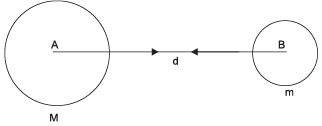Courses

# Gravitation, Science, Class 9 - Test

## 15 Questions MCQ Test Class 9 Science by VP Classes | Gravitation, Science, Class 9 - Test

Description
This mock test of Gravitation, Science, Class 9 - Test for Class 9 helps you for every Class 9 entrance exam. This contains 15 Multiple Choice Questions for Class 9 Gravitation, Science, Class 9 - Test (mcq) to study with solutions a complete question bank. The solved questions answers in this Gravitation, Science, Class 9 - Test quiz give you a good mix of easy questions and tough questions. Class 9 students definitely take this Gravitation, Science, Class 9 - Test exercise for a better result in the exam. You can find other Gravitation, Science, Class 9 - Test extra questions, long questions & short questions for Class 9 on EduRev as well by searching above.
QUESTION: 1

Solution:
QUESTION: 2

Solution:
QUESTION: 3

### A ball is thrown up, the value of ‘g’ will be

Solution:
QUESTION: 4

The mass of body on moon is 40 kg, what is the weight on the earth?

Solution:
QUESTION: 5

The gravitational force between two objects is

Solution:
QUESTION: 6

The gravitation force causes

Solution:
QUESTION: 7

The gravitational force between two objects is F. If masses of both the objects are halved without altering the distance between them, then the gravitational force would become

Solution:
QUESTION: 8

The Earth attracts the moon with a gravitational force of 1020 N. The moon attracts the earth with a gravitational force of

Solution:
QUESTION: 9

The distance between two bodies becomes 6 times more than the usual distance. Then the F becomesSolution:
QUESTION: 10

Newton’s law of gravitation applies to

Solution:
QUESTION: 11

The device used to measure the purity of milk is

Solution:
QUESTION: 12

The cork floats while the nail sinks in the water, this is due to

Solution:
QUESTION: 13

The relative density of silver is 10.8 and the density of water is 103 kg/m2. The density of silver is

Solution:
QUESTION: 14

Buoyant force exerted by different fluids on a given body is

Solution:
QUESTION: 15

Liquid A is denser than liquid B, a body of wood is dipped in both the liquids. The buoyant force experienced by the body in

Solution: